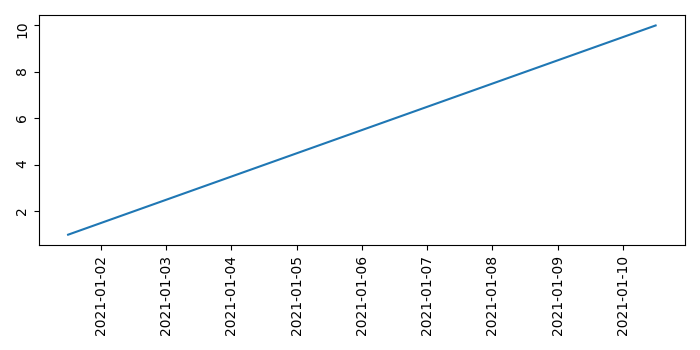# How do I extend the margin at the bottom of a figure in Matplotlib?

To fix the extension of margin at the bottom of a figure, we can take the following steps −

• Using Pandas dataframe, create a df with the keys, time and speed.

• Plot df.time and df.speed using plot() method.

• Tick_params() is a convenience method for changing the appearance of ticks and tick labels. rotation=90 extends the tick labels at the bottom.

• To fix the bottom extension, use tight_layout() method.

## Example

import numpy as np
import pandas as pd
from matplotlib import pyplot as plt
plt.rcParams["figure.figsize"] = [7.00, 3.50]
plt.rcParams["figure.autolayout"] = True
df = pd.DataFrame(dict(time=list(pd.date_range("2021-01-01 12:00:00",
periods=10)), speed=np.linspace(1, 10, 10)))
plt.plot(df.time, df.speed)
plt.tick_params(rotation=90)
plt.show()

## Output